SSC BOARD PAPERS IMPORTANT TOPICS COVERED FOR BOARD EXAM 2024

### Co-ordinate Geometry Class 9th Mathematics Part Ii MHB Solution

##### Co-ordinate Geometry Class 9th Mathematics Part Ii MHB Solution

###### Practice Set 7.1
Question 1.

State in which quadrant or on which axis do the following points lie.

A(-3, 2), B(-5, -2), K(3.5, 1.5),

D(2, 10), E(37, 35), F(15, -18),

G(3, -7), H(0, -5), M(12, 0),

N(0, 9), P(0, 2.5), Q(-7, -3)

The x-y coordinate system is shown below: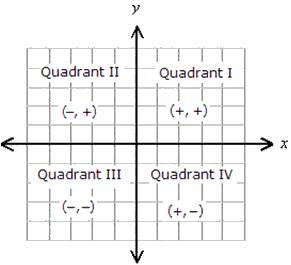The points are calculated as below: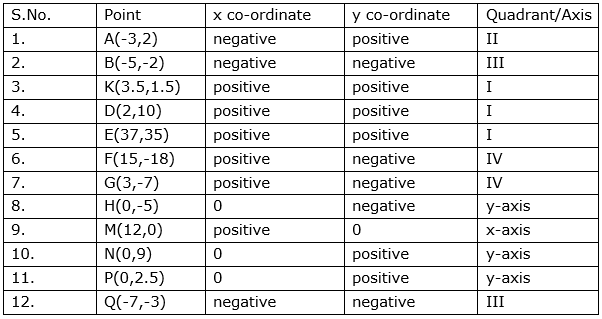Question 2.

In which quadrant are the following points?

i. whose both co-ordinates are positive.

ii. whose both co-ordinates are negative.

iii. whose x co-ordinate is positive, and the y co-ordinate is negative.

iv. whose x co-ordinate is negative and y co-ordinate is positive.

According to co-ordinates Geometry,

i. In First Quadrant, both co-ordinates are positive.

ii.In Third Quadrant, both co-ordinates are negative.

iii.In Fourth Quadrant, x co-ordinate is positive and the y co-ordinate is negative.

iv.In second Quadrant, x co-ordinate is negative and y co-ordinate is positive.

The coordinate-system is shown below: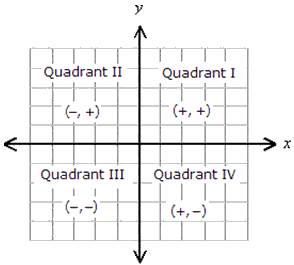Question 3.

Draw the co-ordinate system on a plane and plot the following points.

L(-2, 4), M(5, 6), N(-3, -4), P(2, -3), Q(6, -5), S(7, 0), T(0, -5)

The graph is shown below: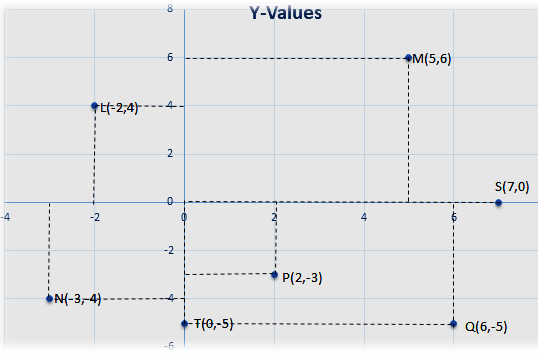Steps for plotting the points:

• Draw X-axis and Y-axis on the plane.

• To find the point M(5,6) draw a line parallel to the Y-axis through the point on X axis which represents the number 5. Through the point on Y-axis which represents the number 6 draw a line parallel to the X-axis .

• The point of intersection of these two lines parallel to the Y and X-axis respectively, is the point M(5,6).

• In the same way, plot the point Q (6,-5),P(2,-3),L(-2,4) and N(-3,-4).

• and the point S(7,0) is lies on X-axis and the point T(0,-5) lies on Y-axis.

###### Practice Set 7.2
Question 1.

On a graph paper plot the points A (3,0), B(3,3), C(0,3). Join A, B and B, C. What is the figure formed?

The graph is shown below: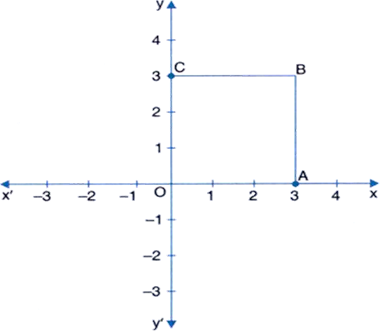Steps are given below:

• O is a centre of XY planes.

• Firstly we have drawn a point A(3,0) which is lies on x-axis and then,

• To find the point B(3,3) draw a line parallel to the Y-axis through the point on X axis which represents the number 3. Through the point on Y-axis which represents the number 3 draw a line parallel to the X-axis .

• And then draw a point C(0,3) is lies on y-axis and,

• Joining a points A,B,C and O then it is formed a SQUARE.

Question 2.

Write the equation of the line parallel to the Y-axis at a distance of 7 units from it to its left.

The equation of line parallel to Y-axis is x = a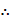a = -7 (because 7 units from left)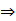x = -7 is required equation.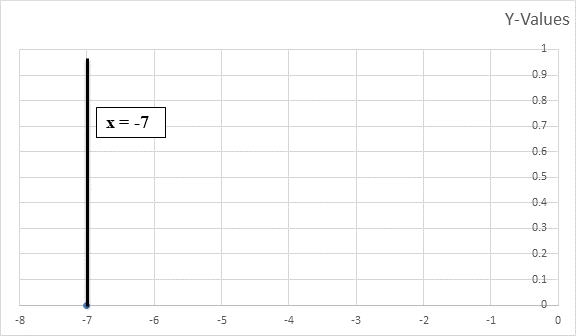X-Values

Steps to draw:

• On a graph paper draw the X-axis and the Y-axis.

• Since it is given that x = -7, draw a line on the left of the Y-axis at a distance of 7 units from it and parallel to it.

Question 3.

Write the equation of the line parallel to the X-axis at a distance of 5 units from it and below the X-axis.

The equation of line parallel to X-axis is y = aa = -5 (because 5 units below the x-axis)y = -5 is required equation.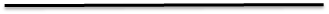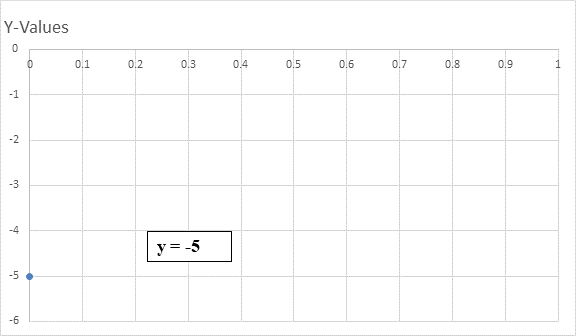Steps:

• On a graph paper draw the X-axis and the Y-axis.

• Since it is given that y = -5, draw a line below the X-axis at a distance of 5 units from it and parallel to it.

Question 4.

The point Q( -3, -2) lies on a line parallel to the Y-axis. Write the equation of the line and draw its graph.

The equation of line parallel to y-axis is x = ax = -3

And the given point is Q(-3,-2)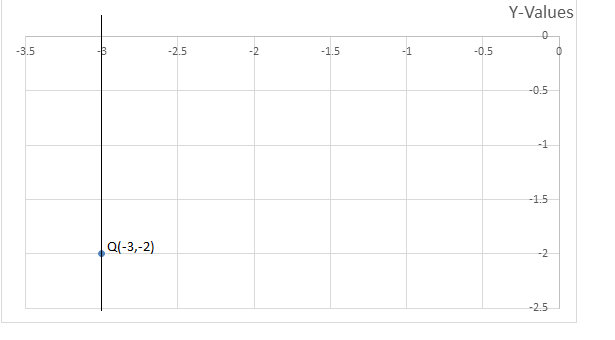Question 5.

Y-axis and line x = -4 are parallel lines. What is the distance between them?

The equation of line parallel to Y-axis is x = a.x = -4a = -4

Since the distance between and the line is 4 units.

The graph is shown below: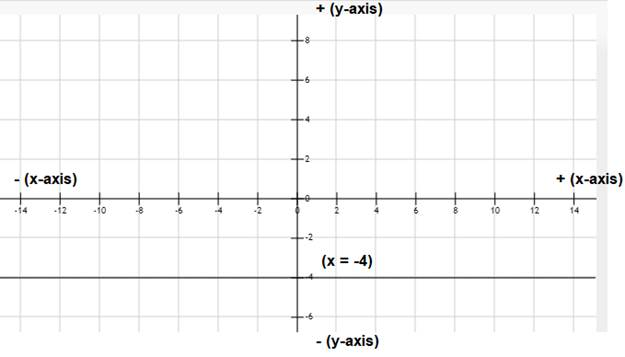Question 6.

Which of the equations given below have graphs parallel to the X-axis, and which ones have graphs parallel to the Y-axis?

i. x=3 ii. y – 2 = 0

iii. x + 6 = 0 iv. Y = -5

i. x = 3x = a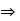The line is parallel to y-axis.

The figure is shown below: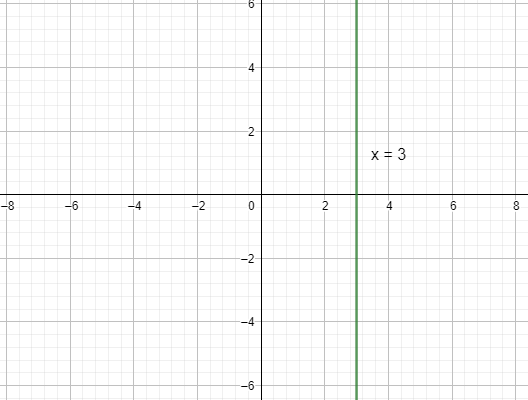ii. y-2 = 0y = 2y = a

The figure is shown below: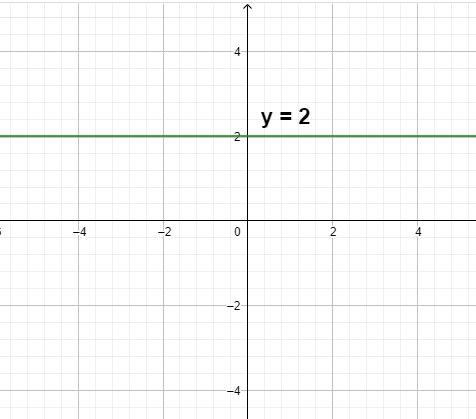And the line is parallel to x-axis.

iii. x + 6 = 0x = -6

The figure is shown below: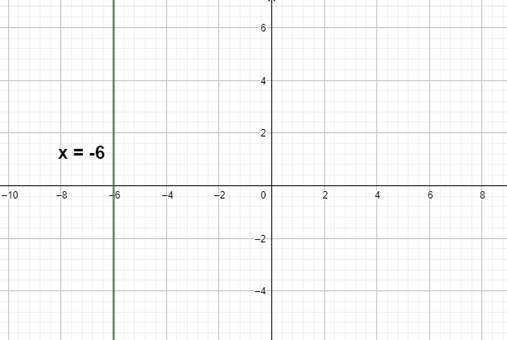And the line is parallel to y-axis.

iv. y = -5y = a

Then, the line is parallel to x-axis.

The figure is shown below: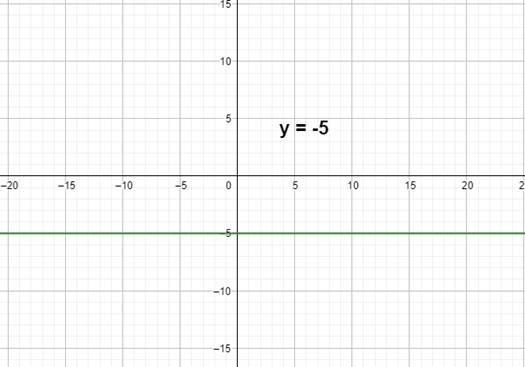Question 7.

On a graph paper, plot the points A(2, 3), B(6, -1) and C(0, 5). If those points are collinear then draw the line which includes them. Write the co-ordinates of the points at which the line intersects the X-axis and the Y-axis.

The figure is shown below: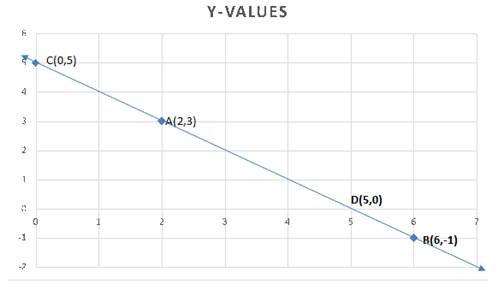• Yes, given points are collinear.

• The line intersects the x-axis at point D(5,0) and the y-axis at point C(0,5).

Question 8.

Draw the graphs of the following equations on the same system of co-ordinates. Write the co-ordinates of their points of intersection.

x + 4 = 0

y - 1 = 0

2x + 3 = 0

3y - 15 = 0

Given equation are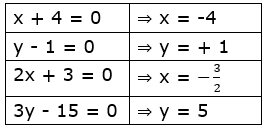The figure is shown below: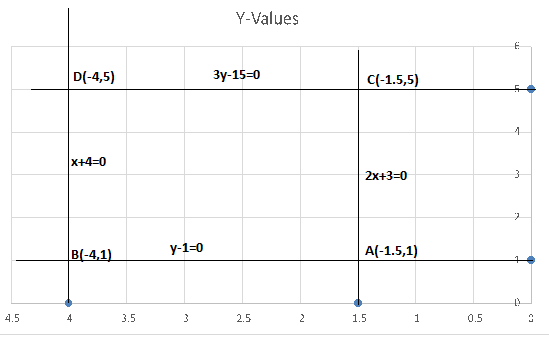• On a graph paper draw the X-axis and the Y-axis.

• Since it is given that x = -4 and x = -1.5, draw a line on the left of the Y-axis at a distance of 4 and 1.5 units from it and parallel to it.

• Since it is given that y = 1 and y = 5, draw a line above the X-axis at a distance of 1and 5 units from it and parallel to it.

• These lines, parallel to the two axes, are the graphs of the given equations.

• And these lines are intersects at the point A(-1.5,1), B(-4,1),C(-1.5,5) and D(-4,5).

Question 9.

Draw the graphs of the equations given below

x + y = 2

x + y = 2

According to the equation,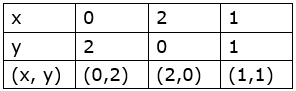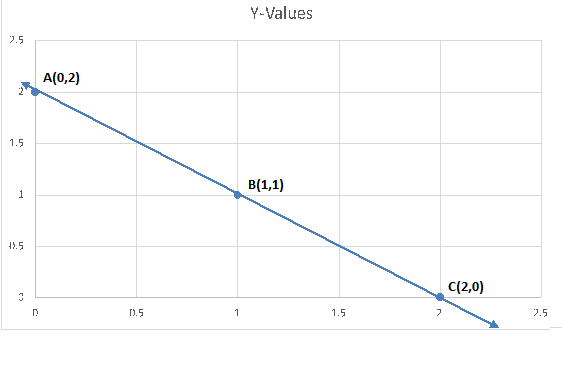• Firstly we have findout the point with the help of given equation.

• Then after plot a xy plane in a coordinate axis.

• The points A,B and C are collinear because all the points lies in the straight line.

Question 10.

Draw the graphs of the equations given below

3x-y = 0

3x-y = 0

According to the given equation,

Now we have find out the points are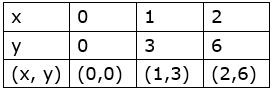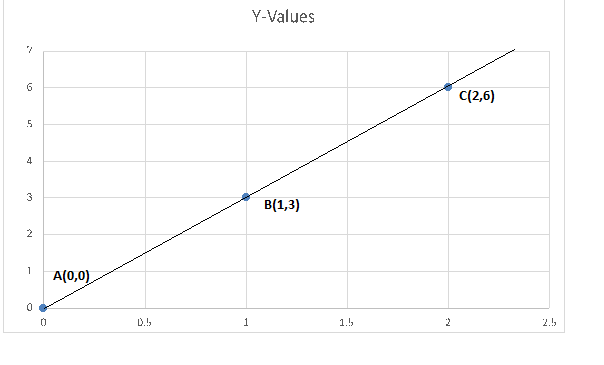• On a graph paper draw the X-axis and the Y-axis.

• Then the points A,B and c are collinear because its lies in a straight line.

Question 11.

Draw the graphs of the equations given below

3x-y = 0

2x + y = 1

According to the given equation,

Now we have find out the points are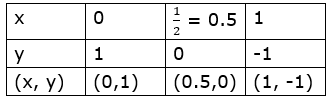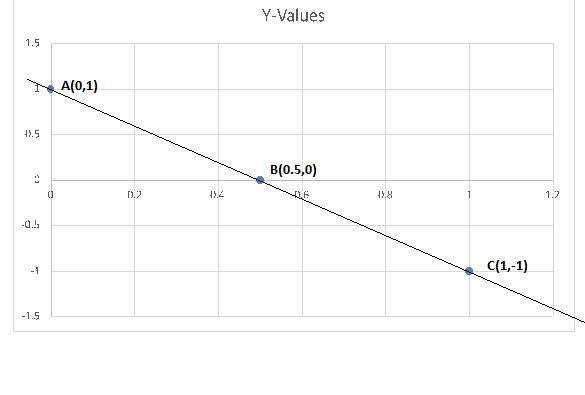• On a graph paper draw the X-axis and the Y-axis.

• Then the points A,B and c are collinear because its lies in a straight line.

###### Problem Set 7
Question 1.

Choose the correct alternative answer for the following questions.

What is the form of co-ordinates of a point on the X-axis?
A. (b,b)

B. (o,b)

C. (a,o)

D. (a,a)

This is because the y co-ordinate point is zero.

Question 2.

Choose the correct alternative answer for the following questions.

Any point on the line y=x is of the form .....
A. (a,a)

B. (o,a)

C. (a,o)

D. (a,-a)y = x

Y = a then x = a.

Question 3.

Choose the correct alternative answer for the following questions.

What is the equation of the X-axis?
A. x =0

B. y =0

C. x+y=0

D. x=y

y = m × x + c

Where c is the intercept of the line on y-axis and m is the gradient of the line. Since intercept of x-axis on y-axis is zero, so c = 0. Gradient of x-axis is also zero because it makes an angle zero with itself. So m = tan(theta) = 0. Putting these values in the general equation we get,

y = 0 × x + 0

y = 0. Thus we get the equation for x-axis as y = 0.

Question 4.

Choose the correct alternative answer for the following questions.

In which quadrant does the point
(-4, -3) lie?
A. First

B. Second

C. Third

D. Fourth

Since both the x & y coordinate are negative. Hence, it lies in the third quadrant.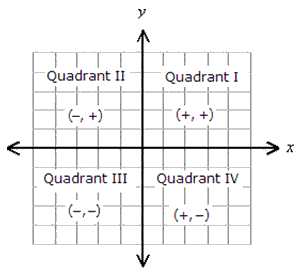Question 5.

Choose the correct alternative answer for the following questions.

What is the nature of the line which includes the points (-5,5), (6,5),
(-3,5), (0,5)?
A. Passes through the origin,

B. Parallel to Y-axis.

C. Parallel to X-axis

D. None of these

All the points are shown below: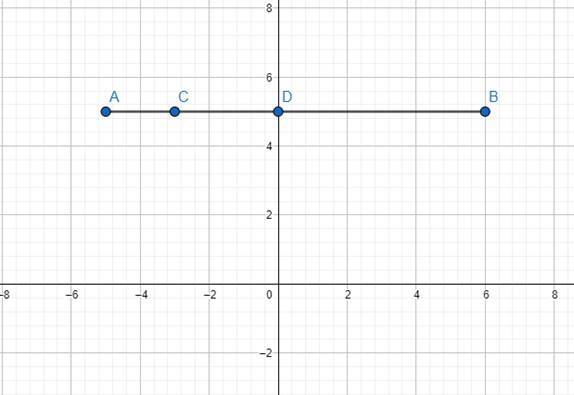Question 6.

Choose the correct alternative answer for the following questions.

Which of the points P (-1,1), Q (3,-4), R(1,-1), S (-2,-3), T (-4,4) lie in the fourth quadrant?
A. P and T

B. Q and R

C. only S

D. P and R

For a point to lie in the 4th quadrant, the x-coordinate should be positive & the y-coordinate should be negative.

As shown in the figure:The figure is given below: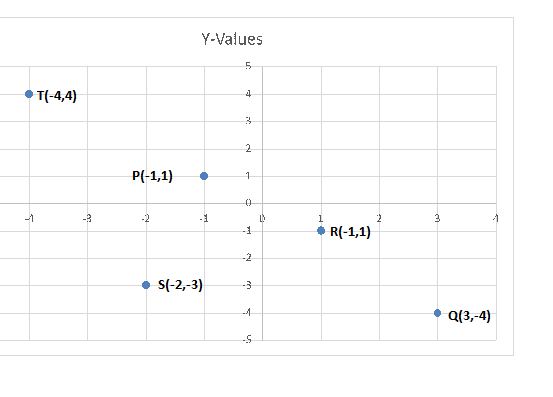Question 7.

Some points are shown in the figure 7.11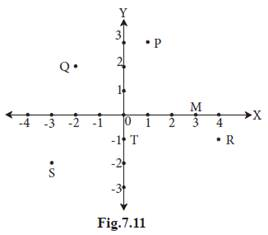With the help of it answer the following questions :

i. Write the co-ordinates of the points Q and R.

ii. Write the co-ordinates of the points T and M.

iii. Which point lies in the third quadrant?

iv. Which are the points whose x and y co-ordinates are equal?

i. The co-ordinates of the points are Q(-2,2) and R(4,-1).

ii. The co-ordinates of the points are T(0,-1) and M(3,0).

iii. The point lies in the third quadrant is S(-3,-2).

iv. Q(-2,2) (both the co-ordinates are equal)

Question 8.

Without plotting the points on a graph, state in which quadrant or on which axis do the following point lie.

i. (5, -3) ii. (-7, -12)

iii. (-23, 4) iv. (-9, 5)

v. (0, -3) vi. (-6, 0)

We should plot the points according to the coordinate system: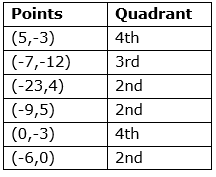Question 9.

Plot the following points on the one and the same co-ordinate system.

A(1, 3), B(-3, -1), C(1, -4),

D(-2, 3), E(0, -8), F(1, 0)

We plot according to the coordinate system: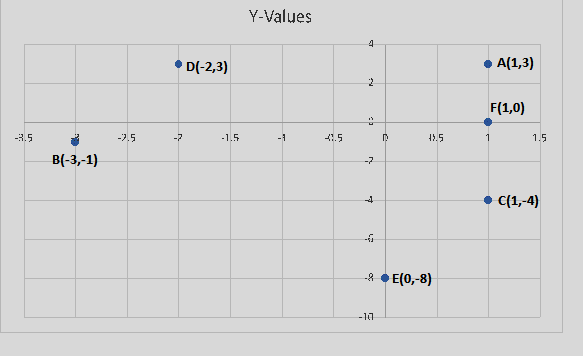Question 10.

In the graph alongside, line LM is parallel to the Y-axis. (Fig. 7.12)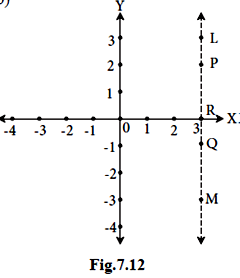i. What is the distance of line LM from the Y-axis?

ii. Write the co-ordinates of the points P, Q and R.

iii. What is the difference between the x co-ordinates of the points L and M?

i.The distance of line LM from the Y-axis is 3.

ii.The co-ordinates of the points are P(3,2),Q(3,-1)and R(3,0).

iii. The difference between the x co-ordinates of the points L and M is 6.

Question 11.

How many lines are there which are parallel to X-axis and having a distance 5 units?

Suppose the required linear equation of a line is y = mx + c

Since, the line is parallel to x-axis, so m = 0

Then equation of the line will be y = c

And this line passes through the point at a distance of 5 units below the x-axis i.e (-5,0)

Then the equation of line is y = -5.

And similarly,

And this line passes through the point at a distance of 5 units above the x-axis i.e (5,0)

Then the equation of line is y = 5.

Question 12.

If ‘a’ is a real number, what is the distance between the Y-axis and the line x = a?